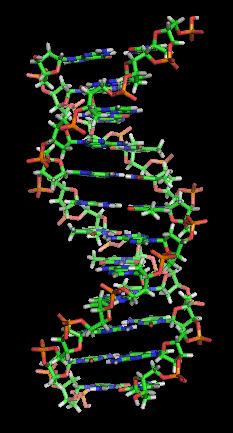## Tuesday, March 24, 2015

### On the origin of the helix structure from the view point of GMS

Helix structure has been prevalent in biological world. It can be fund in small scale like the folding of DNA and protein sequences, and in large scale like plants.Whereas the mathematical description of the helix structure is clear; the mechanism that gives rise to such structure is not so obvious. Do all those structure share a common mechanism? Why don't they show up in inorganic world?  This note tries to demonstrate with GMS samples that helix structure comes about by some simple dynamics rooted in the discrete sequence structure;

Recall that GMS produces a sequence of high dimensional vectors from a discrete sequences; and the high dimensional vectors are embedded in to low dimensional space according their affinity defined in the following affinity function:

Where t>t' are the timestamps of two vectors produced at type t and t'. We notice that the affinity function consists of two parts:andThe first part is only dependent on the timestamp: it reduces the affinity calculated by the second part exponentially depending on time separating these two vectors. It is the second part that accounts for variations in the sequence, si.

In order the see the effect of decay in time dimension, I modified the loopy GMS algorithm so that the affinity function only contains the first part while the second part (the sequence dependent part) is set to the constant 1.0. I ran the algorithm with following parameters: n=25000; K=10; the sequence is the constant sequence with 100 copies of the letter 'A'; the decay speed λ is successively set to 0.125, 0.25, 0.5 and 1.0. The following screen cast shows the resulting corresponding GMS maps in 3D space:

These maps show clearly the helix alike structure; and the winding number goes up as the as decay speed λ goes higher.

To verify that it is the exponential decaying that leads to helix alike structure, I have replaced the affinity function with three different "decaying" functions: Δt-2, Δt-1 and Δt-0.5 with Δt := t-t'. The following pictures shows the corresponding GMS maps:

We can see clearly that these decay functions result in structures that are totally different from the helix structure. Thus, these simulations indicate that the exponential decay of affinity plays a significant role in forming the helix structure.

We notice that if the scanning size K is sufficiently large and the sequence is random, the affinity contribution of the second (sequence dependent) part will, more or less, become constant. Thus, the helix structure may serve as model for completely random sequences. From this point of view, we might call the helix structure the no-information base model. Additional information in sequences should manifest in discrepancy between their GMS maps and the helix structure.

Seeing some π symbols in some formula, the great physicist Richard Fynman once asked: Where is the circle?  In terms of modern genetics, his remark basically assumes that π  is the "gene" for the circle as a phenotype feature. Many such analytical "genes" are carried forward and spread around in various fields, they manifest in different forms, but always keep their intrinsic unchanged.  Here, this note tried to demonstrate that the "gene" for helix structure is the exponential decay of affinity.